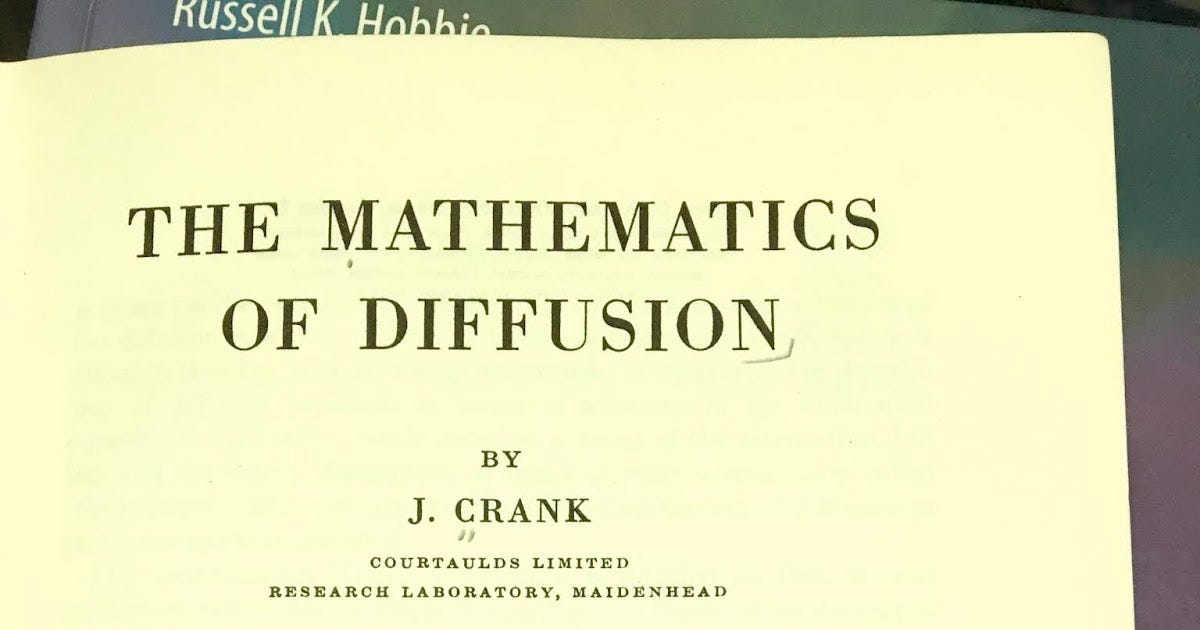# Diffusion with a BufferThe Mathematics of Diffusion, by John Crank.

Homework Problem 27 in Chapter 4 of Intermediate Physics for Medicine and Biology examines diffusion in the presence of a buffer. The problem shows that the buffer slows diffusion and introduces the idea of an effective diffusion constant. I like this problem but I admit it’s rather long-winded. Recently, when thumbing through John Crank’s book (doesn’t everyone thumb through The Mathematics of Diffusion on occasion?), I found an easier way to present the same basic idea. Below is a simplified version of Problem 27.

Section 4.8

Problem 27½. Calcium ions with concentration C diffuse inside cells. Assume that this free calcium is in instantaneous local equilibrium with calcium of concentration S that is bound to an immobile buffer, such that

S = RC , (1)

where R is a dimensionless constant. Calcium released from the buffer acts as a source term in the diffusion equation

∂C/∂t = D ∂^2C/∂x^2 − ∂S/∂t . (2)

(a) Explain in words why ∂S/∂t is the correct source term. Be sure to address why there is a minus sign.

(b) Substitute Eq. (1) into Eq. (2), derive an equation of the form

C/∂t = D_eff ∂^2C/∂x^2 , (3)

and obtain an expression for the effective diffusion constant D_eff.

(c) If R is much greater than one, describe the physical affect the buffer has on diffusion.

(d) Show that this problem corresponds to the case of Problem 27 when [ B] is much greater than [ CaB]. Explain physically what this means.

To do part (d), you will need to look at the problem in IPMB.

The bottom line: an immobile buffer hinders diffusion. The stronger the buffer (the larger the value of R), the slower the calcium diffuses. The beauty of the homework problem is that it illustrates this property with only a little mathematics.

Enjoy!

Originally published at http://hobbieroth.blogspot.com.

--

--